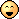Mis à jour le 04/10/2021

## Manipulate data contained in DataFrames

Connectez-vous ou inscrivez-vous gratuitement pour bénéficier de toutes les fonctionnalités de ce cours !Now that you know how to create a DataFrame, let's look at some other common data operations. To do this, I suggest you use a DataSet available in the Seaborn library! The dataset in question includes data on the survivors of the Titanic.

In this chapter, we'll follow a "typical" working session.

``````import numpy as np
import pandas as pd
import seaborn as sns
``````

The first thing to do is to take a quick look at our data.

``````titanic.head()
``````

Now, try looking at all ages. The  `unique`  function returns the unique values present in a Pandas data structure.

``````titanic.age.unique()
``````
`array([22. , 38. , 26. , 35. , nan, 54. , 2. , 27. , 14. , 4. , 58. , 20. , 39. , 55. , 31. , 34. , 15. , 28. , 8. , 19. , 40. , 66. , 42. , 21. , 18. , 3. , 7. , 49. , 29. , 65. , 28.5 , 5. , 11. , 45. , 17. , 32. , 16. , 25. , 0.83, 30. , 33. , 23. , 24. , 46. , 59. , 71. , 37. , 47. , 14.5 , 70.5 , 32.5 , 12. , 9. , 36.5 , 51. , 55.5 , 40.5 , 44. , 1. , 61. , 56. , 50. , 36. , 45.5 , 20.5 , 62. , 41. , 52. , 63. , 23.5 , 0.92, 43. , 60. , 10. , 64. , 13. , 48. , 0.75, 53. , 57. , 80. , 70. , 24.5 , 6. , 0.67, 30.5 , 0.42, 34.5 , 74. ])`

I should also mention the excellent  `describe`  function. It provides various statistics (average, maximum, minimum, etc.) on the data in each column:

``````titanic.describe(include="all")
``````

We use the  `include="all"`  to include non-numeric columns in our analysis. This function results in lots of very useful data about the distribution of our data (minimum, maximum, average, etc.)

You might have noticed  `NaN` values in the `describe`  function.  NaN literally stands for Not a Number and is used to represent a value that is undefined or unrepresentable. For example, we obtain  `NaN`  if we ask Pandas to calculate the average of a column of text.

In fact, the result of any operation involving a  `NaN`  is, in turn, a  `NaN`For example, if one of your columns contains a  `NaN`  (because the true value is not known), the result of all arithmetic operations involving this value (such as the column’s average) will be  `NaN` , unless you avoid taking this value into account. Pandas can do this, as can be seen in our age column example above.

Dealing with missing values is covered in another course. Here, we will look at two operations you can apply to`NaN`.

The first is to replace  `NaN`  with other values. This operation is performed using the  `fillna`  function. Let's look at its application on the `age` column:

``````titanic.age.head(10)
``````
```0 22.01 38.02 26.03 35.04 35.05 NaN 6 54.07 2.08 27.09 14.0
Name: age, dtype: float64```
``````titanic.fillna(value={"age": 0}).age.head(10)
``````

This returns a DataFrame where all  `NaN`  in the age column have been replaced by 0.

```0 22.01 38.02 26.03 35.04 35.05 0.0 6 54.07 2.08 27.09 14.0
Name: age, dtype: float64```

We could also have filled the  `NaN`  with the previous values:

``````titanic.fillna(method="pad").age.head(10)
``````
```0 22.01 38.02 26.03 35.04 35.05 35.0 6 54.07 2.08 27.09 14.0
Name: age, dtype: float64```

Secondly, the  `dropna`  function let's you delete axes (columns or rows) that contain  `NaN`  . By default, it deletes the relevant lines:

``````titanic.dropna().head(10)
``````

But we can also delete the columns altogether!

``````titanic.dropna(axis="columns").head()
``````

Use the `rename`  function to rename the columns or rows of a DataFrame. You can do this in two ways.

``````titanic.rename(columns={"sex":"gender"})
``````

Renames the column "sex" to "gender". While:

``````f = lambda x: x+1
titanic.rename(index=f)
``````

applies the function `f` to all columns.

The  `drop`  function allows you to delete axes (columns or rows) from a DataFrame.

``````titanic.drop(0)
``````

Will delete the line with an index equal to 0.

``````titanic.drop(columns=["age"])
``````

Deletes the "age" column.

Before you get into relational algebra, let's have a look at pivot tables. You may be familiar with this concept if, for example you have used them in spreadsheet software. These tables are used to synthesize the data in a DataFrame. Again, let's use our Titanic dataset as an example.

To see the distribution of survivors by gender and ticket type, we only need one line:

``````titanic.pivot_table('survived', index='sex', columns='class')
``````

The result is perfectly understandable:

By default,  `pivot_table`  groups the data according to the criteria we specify, and aggregates the results on average. We can also specify other functions. For example, if we want to know the total number of survivors in each case, we can use the sum function.

``````titanic.pivot_table('survived', index='sex', columns='class', aggfunc="sum")
``````

`pivot_table`  is a very powerful function that also allows for multi-level aggregations. For example, we can see the age of survivors as an additional dimension. As the exact number of years is of little interest to us, we can group the ages into three categories, thanks to the  `cut`  function.

``````titanic.dropna(inplace=True)
age = pd.cut(titanic['age'], [0, 18, 80])
titanic.pivot_table('survived', ['sex', age], 'class')
``````

The result is a multi-indexed DataFrame:

Notice the two levels of indexation on the left.

On a DataFrame, you can:

• Replace missing data using the `fillna`  function

• Rename columns using  `rename`

• Delete axes using `drop`

• Perform multi-level aggregations using  `pivot_table`

There you go. I hope you have a better idea of Pandas' capabilities. In the next chapter, you will see how to perform linear algebra operations (as in SQL language) on DataFrames!